# NCERT Solutions for Class 9 Maths Chapter 7 Exercise 7.2 – Triangles

Download NCERT Solutions for Class 9 Maths Chapter 7 Exercise 7.2 – Triangles. This Exercise contains 8 questions, for which detailed answers have been provided in this note. In case you are looking at studying the remaining Exercise for Class 9 for Maths NCERT solutions for Chapter 7 or other Chapters, you can click the link at the end of this Note.

### NCERT Solutions for Class 9 Maths Chapter 7 Exercise 7.2 – Triangles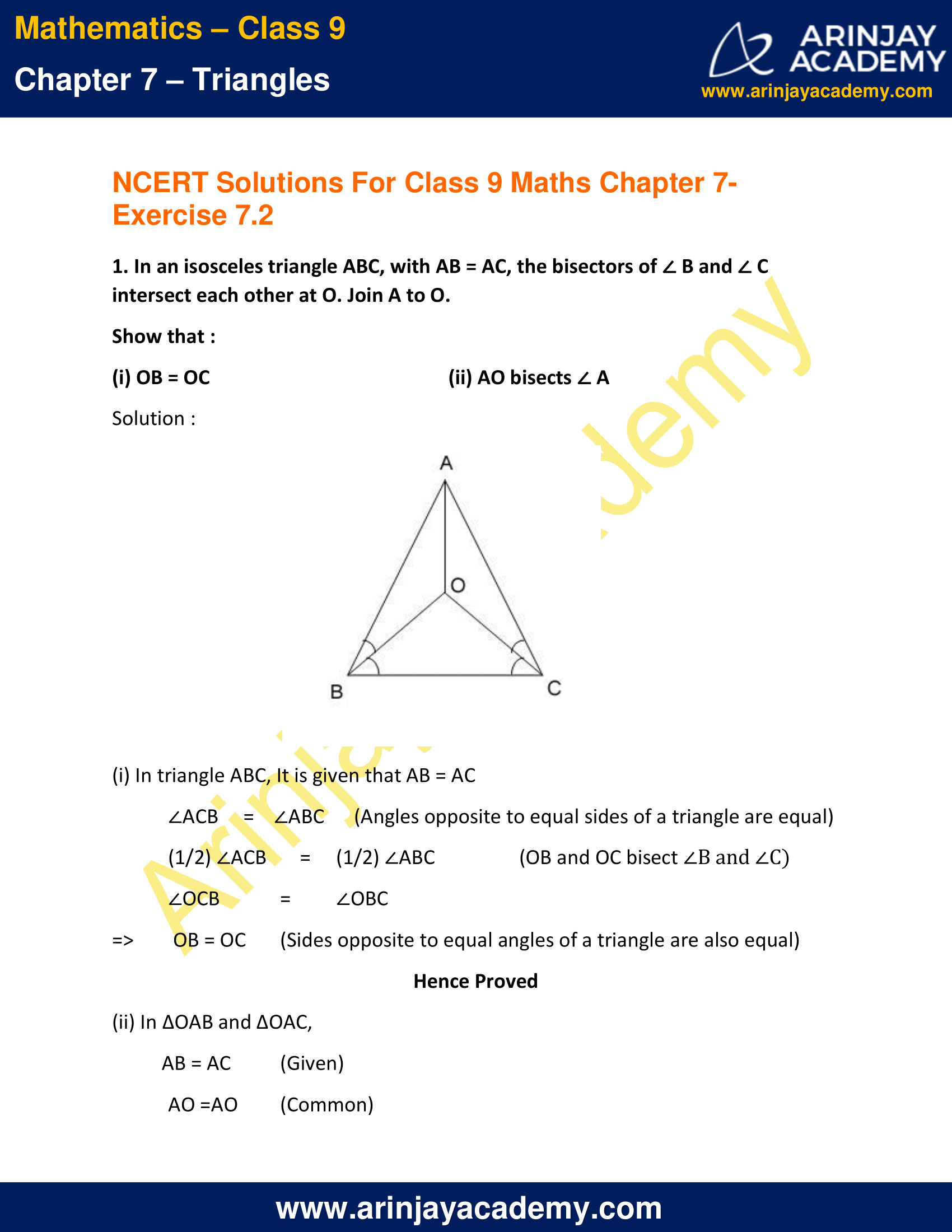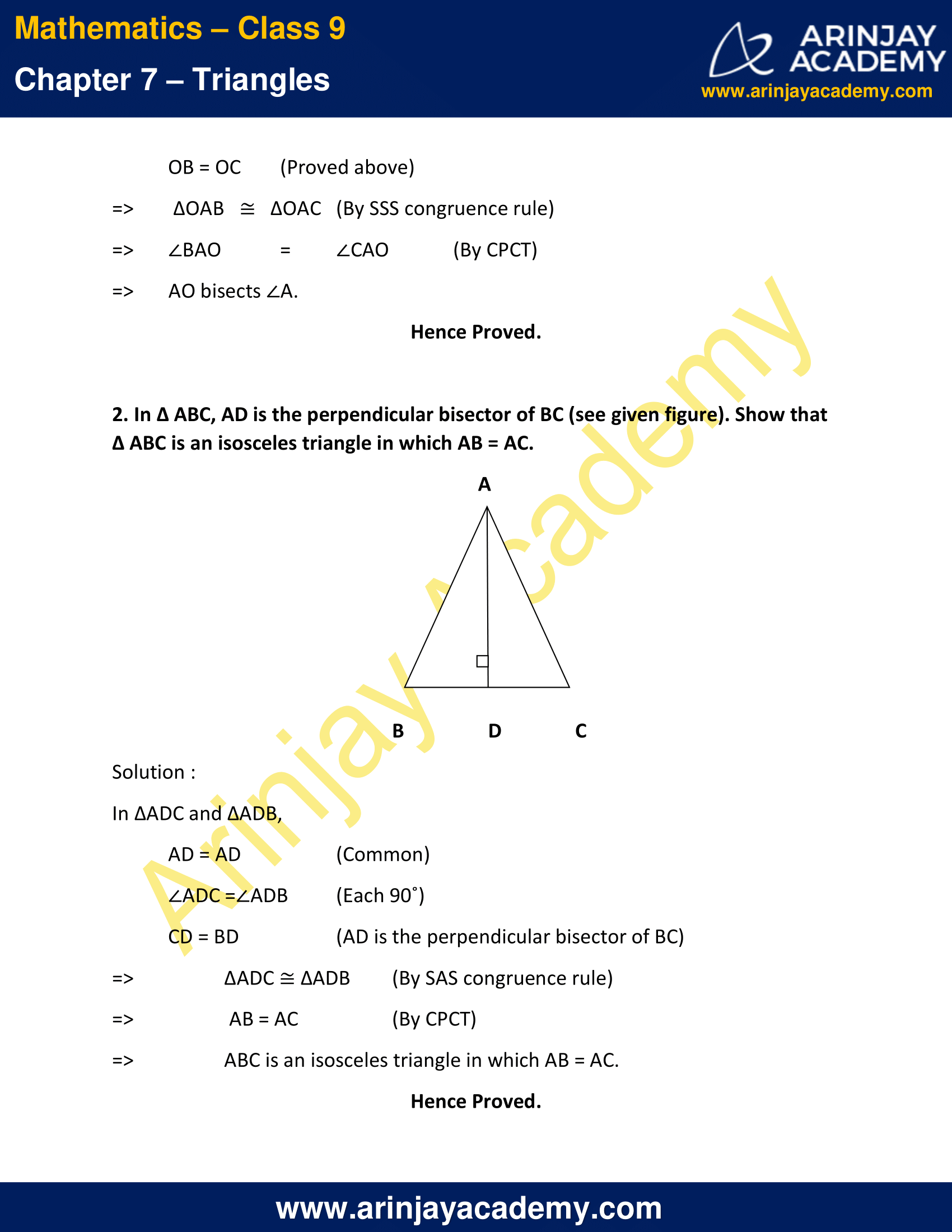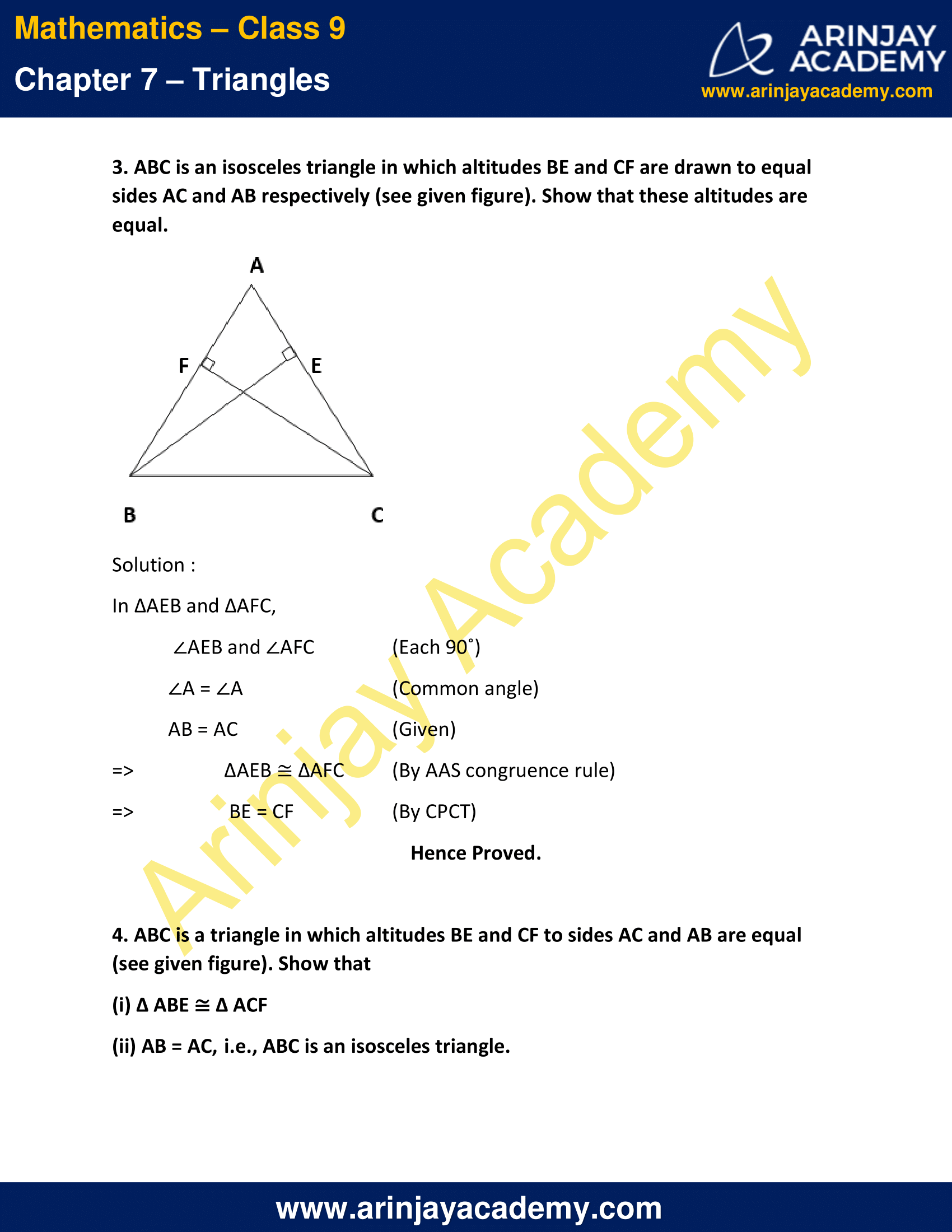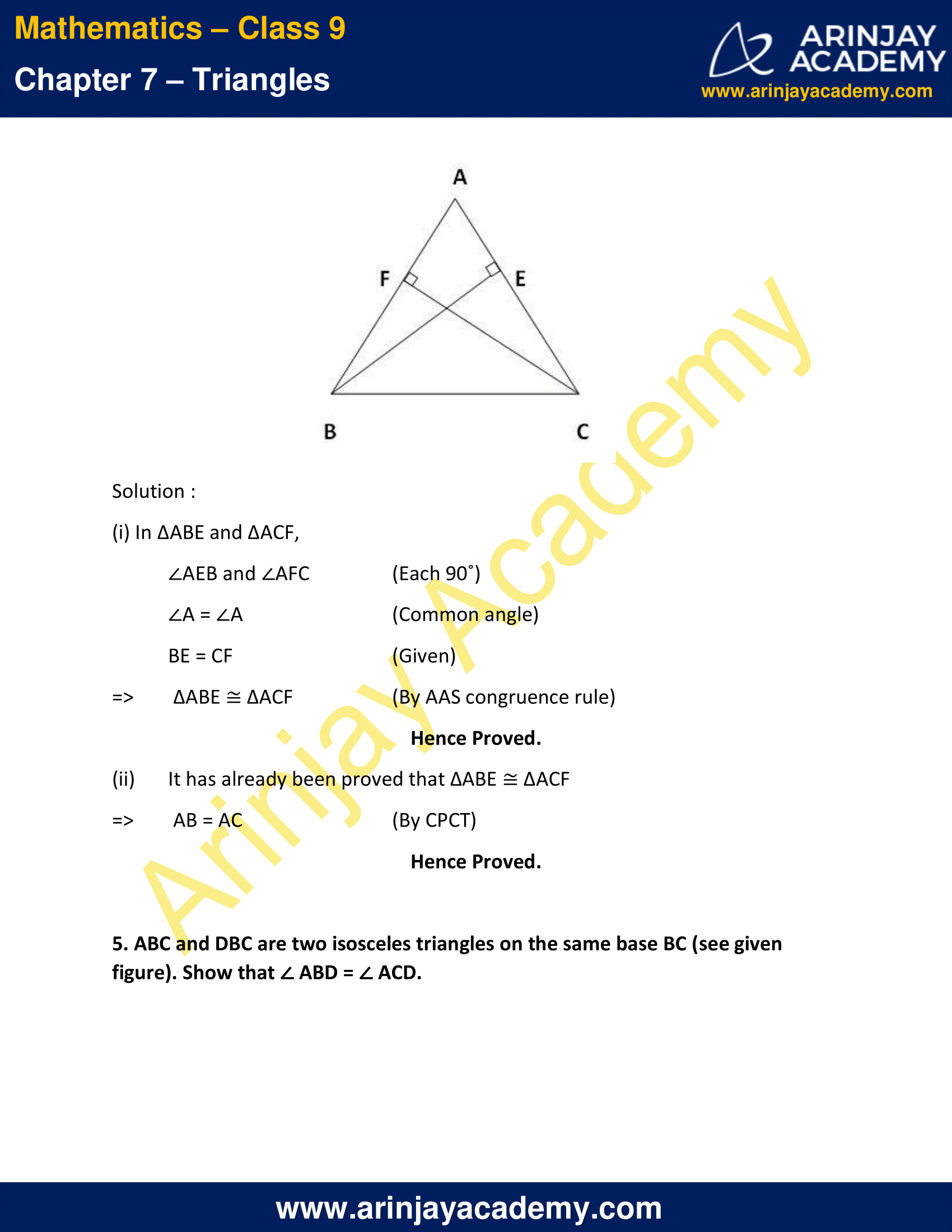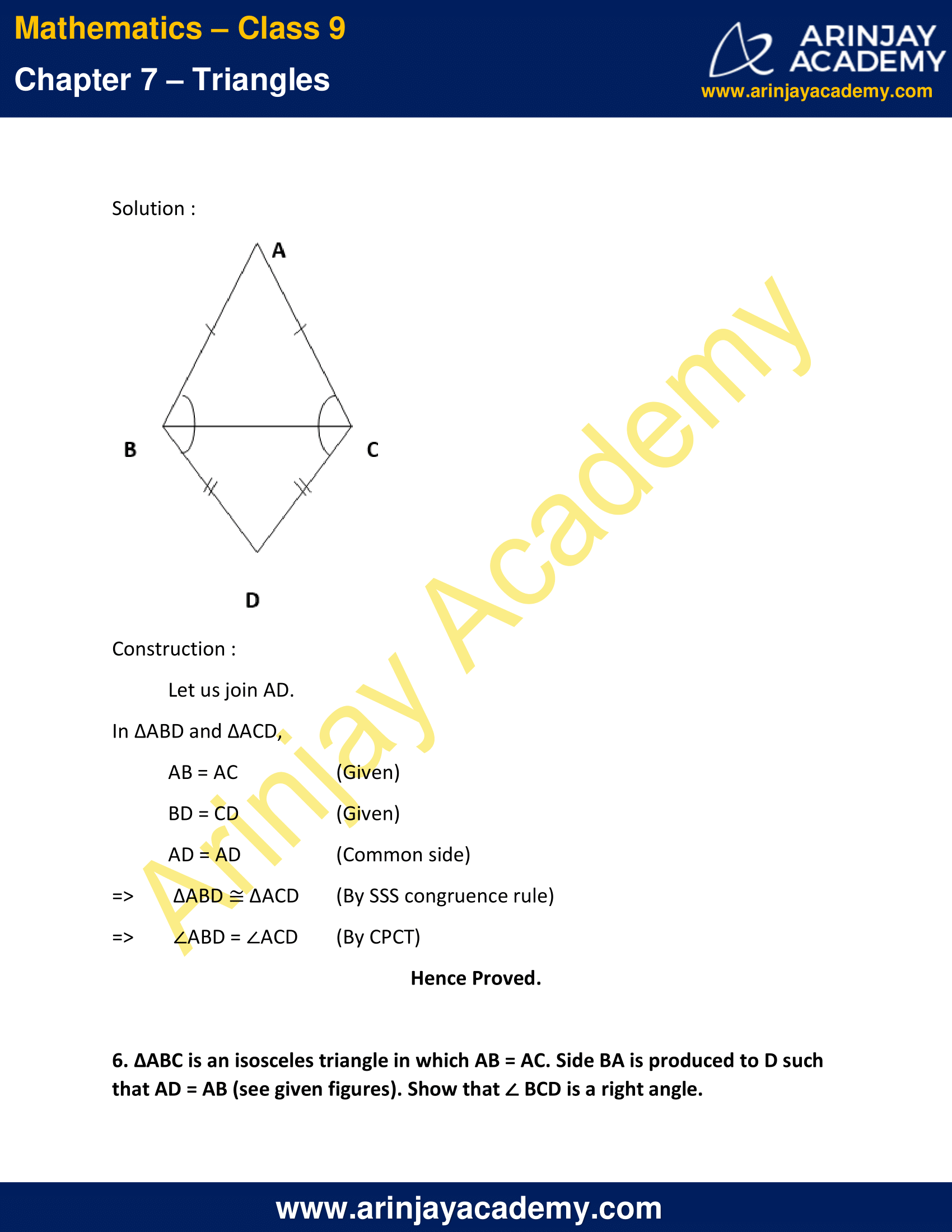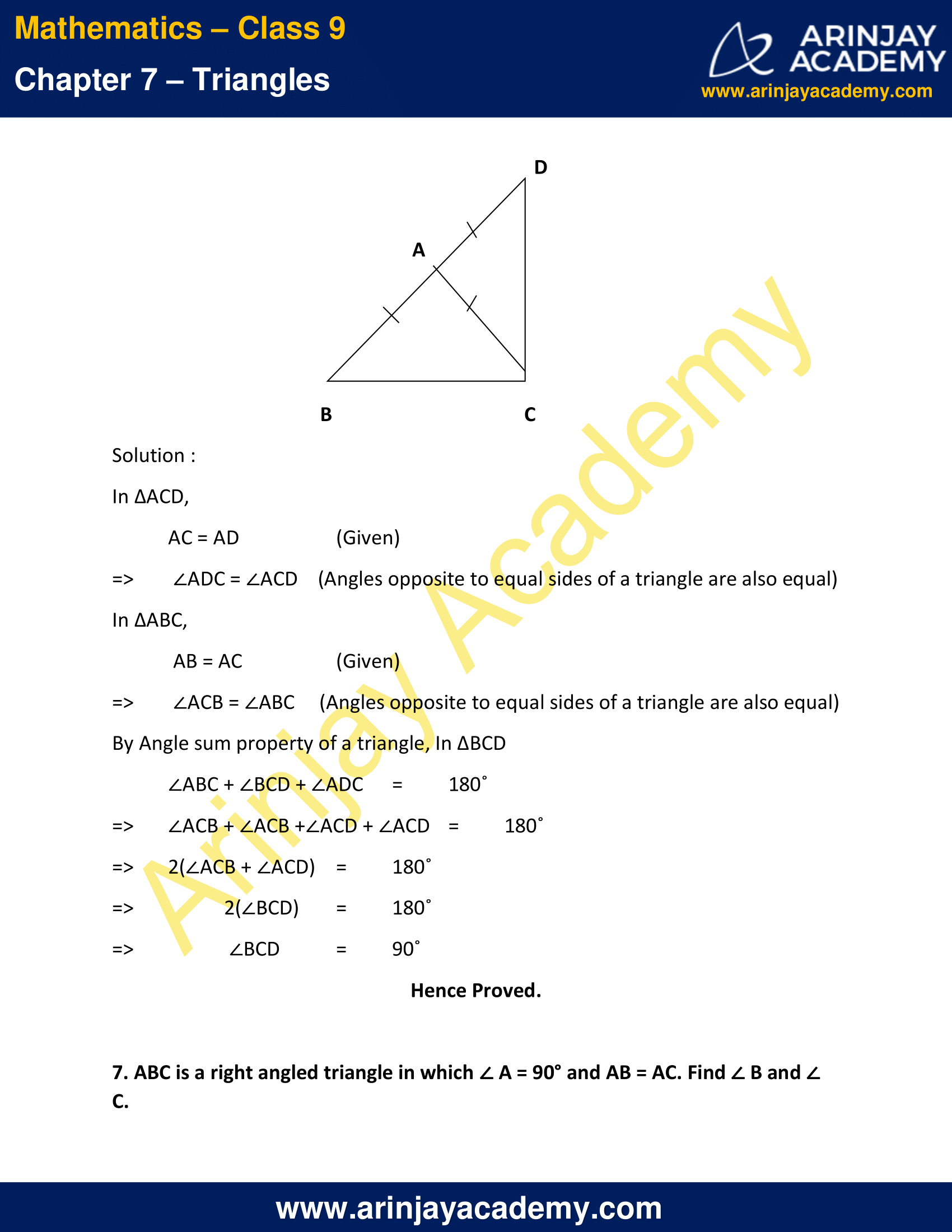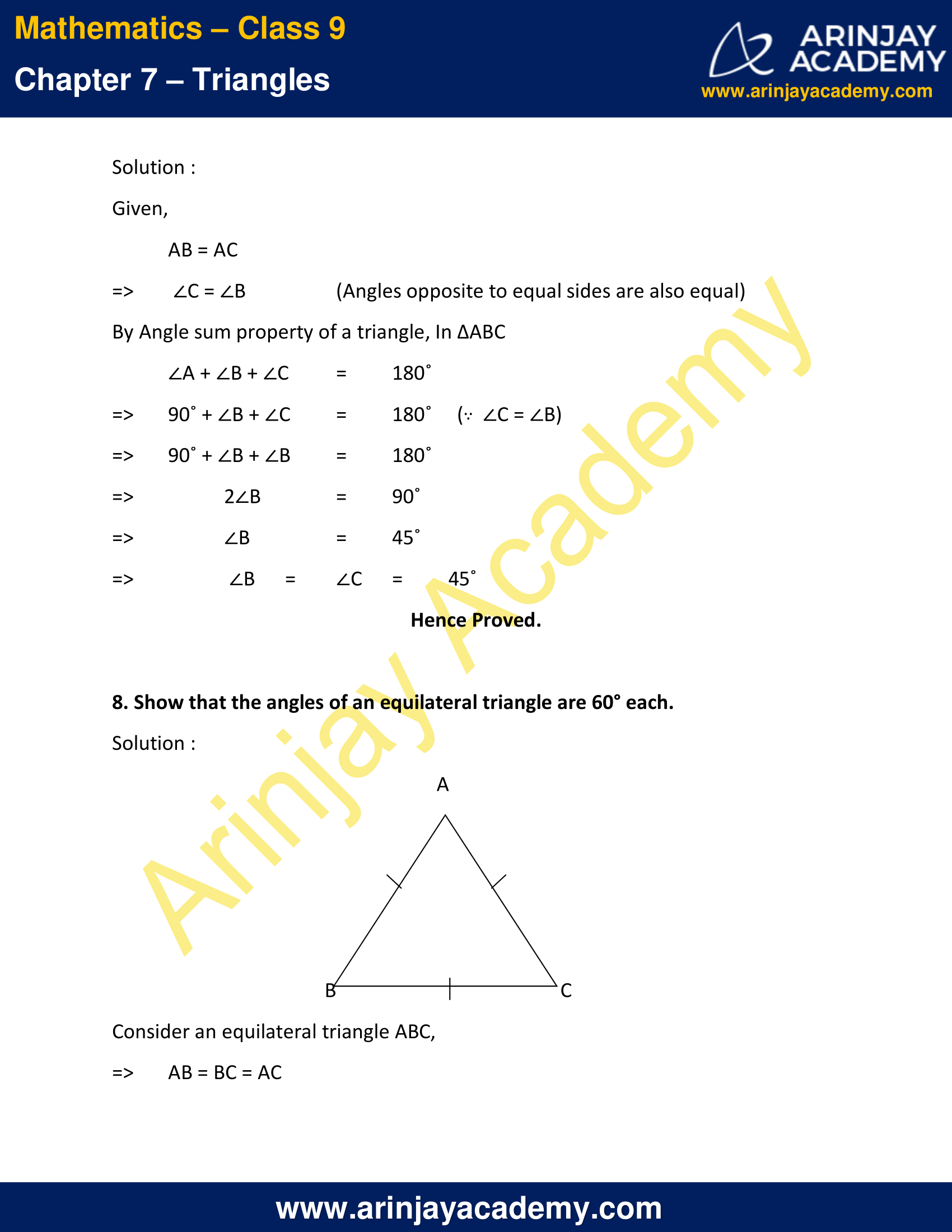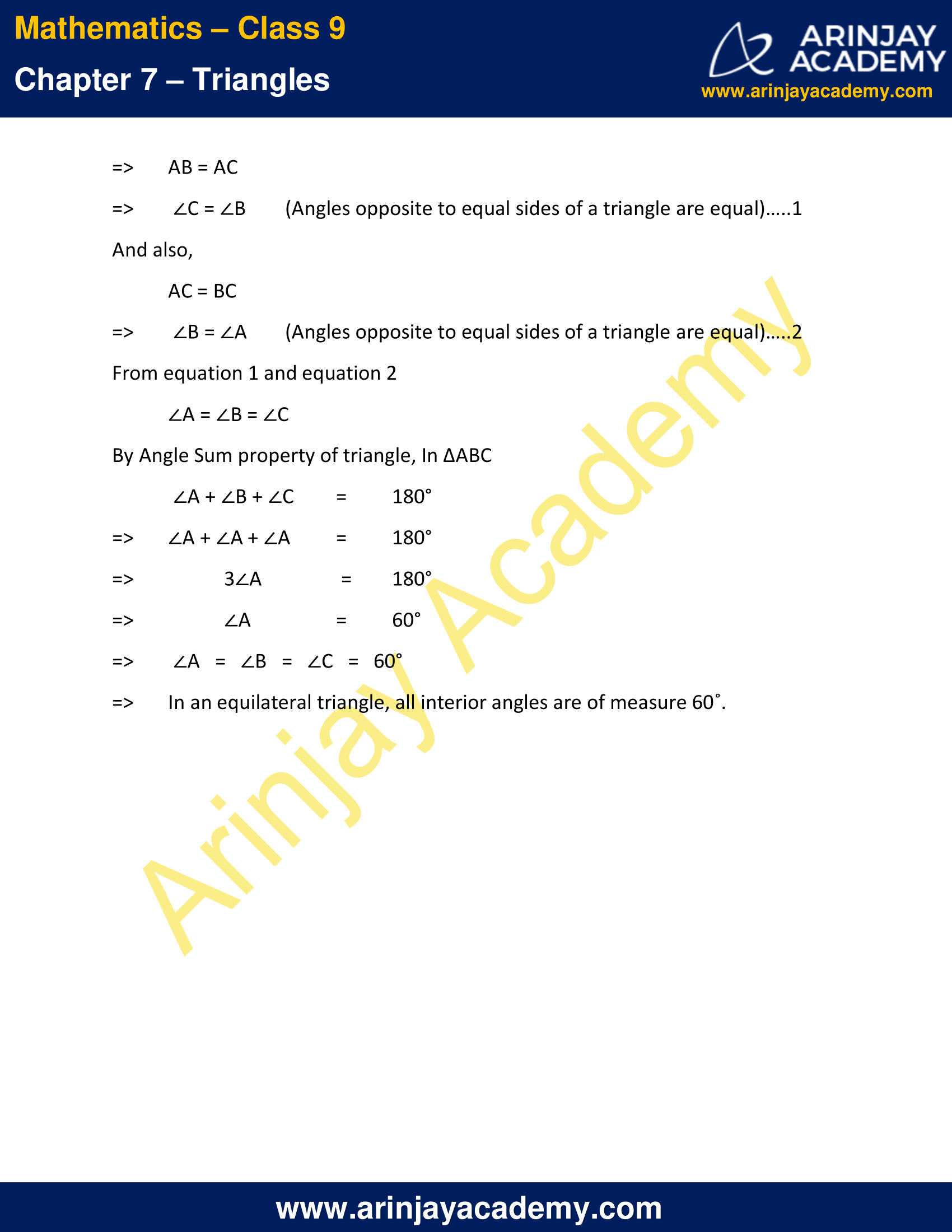NCERT Solutions for Class 9 Maths Chapter 7 Exercise 7.2 – Triangles

1. In an isosceles triangle ABC, with AB = AC, the bisectors of B and C intersect each other at O. Join A to O.
Show that :
(i) OB = OC
(ii) AO bisects
A

Solution :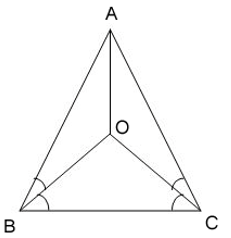(i) In triangle ABC, It is given that AB = AC
∠ACB = ∠ABC   (Angles opposite to equal sides of a triangle are equal)
(1/2) ∠ACB = (1/2) ∠ABC  (OB and OC bisect ∠B and ∠C)
∠OCB = ∠OBC
=> OB = OC   Sides opposite to equal angles of a triangle are also equal)
Hence Proved

(ii) In ∆OAB and ∆OAC,
AB = AC  (Given)
AO =AO  (Common)
OB = OC  (Proved above)
=> ∆OAB   ≅   ∆OAC   (By SSS congruence rule)
=> ∠BAO  =  ∠CAO  (By CPCT)
=>  AO bisects ∠A.
Hence Proved.

2. In ∆ ABC, AD is the perpendicular bisector of BC (see given figure). Show that ∆ ABC is an isosceles triangle in which AB = AC.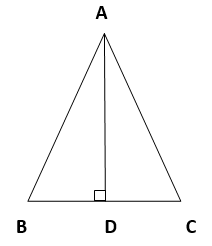Solution :

In ∆ADC and ∆ADB,
AD = AD   (Common)
∠ADC =∠ADB  (Each 90˚)
CD = BD   (AD is the perpendicular bisector of BC)
=>  ∆ADC ≅ ∆ADB  (By SAS congruence rule)
=>  AB = AC  (By CPCT)
=>  ABC is an isosceles triangle in which AB = AC.
Hence Proved.

3. ABC is an isosceles triangle in which altitudes BE and CF are drawn to equal sides AC and AB respectively (see given figure). Show that these altitudes are equal.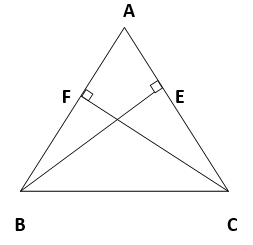Solution :

In ∆AEB and ∆AFC,
∠AEB and ∠AFC   (Each 90˚)
∠A = ∠A    (Common angle)
AB = AC     (Given)
=>  ∆AEB ≅ ∆AFC   (By AAS congruence rule)
=>  BE = CF   (By CPCT)
Hence Proved.

4. ABC is a triangle in which altitudes BE and CF to sides AC and AB are equal (see given figure). Show that
(i) ∆ ABE ∆ ACF
(ii) AB = AC, i.e., ABC is an isosceles triangle.Solution :

(i) In ∆ABE and ∆ACF,
∠AEB and ∠AFC  (Each 90˚)
∠A = ∠A   (Common angle)
BE = CF   (Given)
=> ∆ABE ≅ ∆ACF  (By AAS congruence rule)
Hence Proved.

(ii) It has already been proved that ∆ABE ≅ ∆ACF
=>  AB = AC   (By CPCT)
Hence Proved.

5. ABC and DBC are two isosceles triangles on the same base BC (see given figure). Show that ABD = ACD.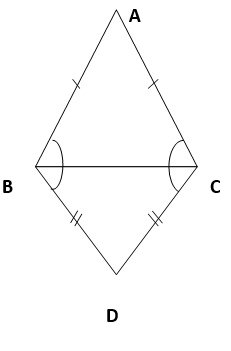Solution :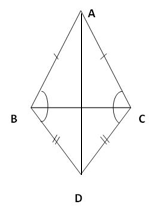Construction :
Let us join AD.
In ∆ABD and ∆ACD,
AB = AC   (Given)
BD = CD   (Given)
AD = AD   (Common side)
=> ∆ABD ≅ ∆ACD  (By SSS congruence rule)
=> ∠ABD = ∠ACD   (By CPCT)
Hence Proved.

6. ∆ABC is an isosceles triangle in which AB = AC. Side BA is produced to D such that AD = AB (see given figures). Show that BCD is a right angle.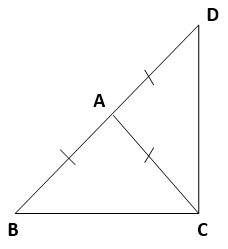Solution :

In ∆ACD,
AC = AD   (Given)
=> ∠ADC = ∠ACD    (Angles opposite to equal sides of a triangle are also equal)
In ∆ABC,
AB = AC   (Given)
=> ∠ACB = ∠ABC   (Angles opposite to equal sides of a triangle are also equal)
By Angle sum property of a triangle, In ∆BCD
∠ABC + ∠BCD + ∠ADC = 180˚
=> ∠ACB + ∠ACB +∠ACD + ∠ACD = 180˚
=>  2(∠ACB + ∠ACD) = 180˚
=>  2(∠BCD) = 180˚
=>  ∠BCD = 90˚
Hence Proved.

7. ABC is a right angled triangle in which A = 90° and AB = AC. Find B and C.

Solution :

Given,
AB = AC
=> ∠C = ∠B  (Angles opposite to equal sides are also equal)
By Angle sum property of a triangle, In ∆ABC
∠A + ∠B + ∠C = 180˚
=> 90˚ + ∠B + ∠C = 180˚     (∵  ∠C = ∠B)
=> 90˚ + ∠B + ∠B = 180˚
=> 2∠B = 90˚
=> ∠B = 45˚
=> ∠B = ∠C = 45˚
Hence Proved.

8. Show that the angles of an equilateral triangle are 60° each.

Solution :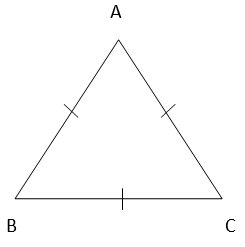Consider an equilateral triangle ABC,
=> AB = BC = AC
=> AB = AC
=> ∠C = ∠B (Angles opposite to equal sides of a triangle are equal)…..1
And also,
AC = BC
=> ∠B = ∠A (Angles opposite to equal sides of a triangle are equal)…..2
From equation 1 and equation 2
∠A = ∠B = ∠C
By Angle Sum property of triangle, In ∆ABC
∠A + ∠B + ∠C = 180°
=> ∠A + ∠A + ∠A = 180°
=> 3∠A = 180°
=> ∠A = 60°
=> ∠A   =   ∠B   =   ∠C   =   60°
=> In an equilateral triangle, all interior angles are of measure 60º.

NCERT Solutions for Class 9 Maths Chapter 7 Exercise 7.2 – Triangles, has been designed by the NCERT to test the knowledge of the student on the topic – Some Properties of a Triangle

• Angles opposite to equal sides of an isosceles triangle are equal
• The sides opposite to equal angles of a triangle are equal.

The next Exercise for NCERT Solutions for Class 9 Maths Chapter 7 Exercise 7.3 – Triangles can be accessed by clicking here.

Download NCERT Solutions for Class 9 Maths Chapter 7 Exercise 7.2 – Triangles